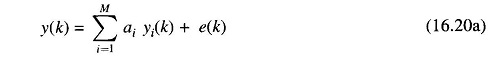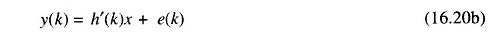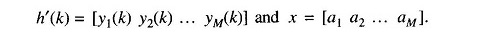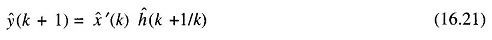## Long Term Load Forecasting in Power System:

Long Term Load Forecasting – If the load forecasts are required for planning purposes, it is necessary to select the lead time to lie in the range of a few months to a few years. In such cases, the load demand should be decomposed in a manner that reflects the dependence of the load on the various segments of the economy of the concerned region. For example, the total load demand y(k) may be decomposed aswhere ai are the regression coefficients, yi(k) are the chosen economic variables and e(k) represents the error of modelling. A relatively simple procedure is to rewrite the model equation in the familiar vector notationwhereThe regression coefficients may then be estimated using the convenient least squares algorithm. The load forecasts are then possible through the simple relationwhere x̂(k) is the estimate of the coefficient vector based on the data available till the kth sampling point and ĥ(k + 1/k) is the one-step-ahead prediction of the regression vector h(k).